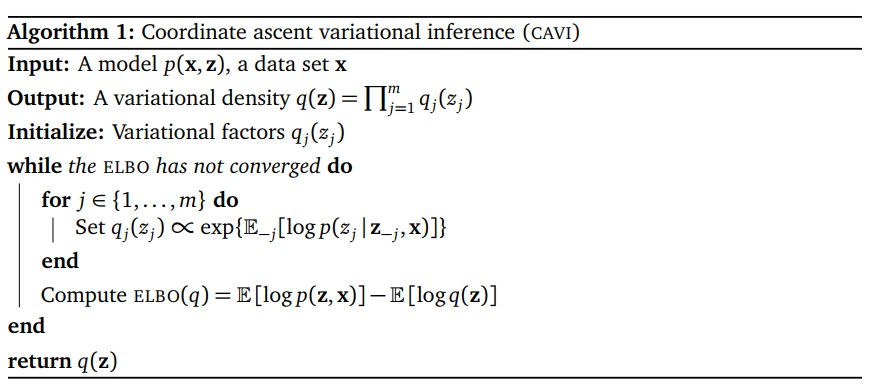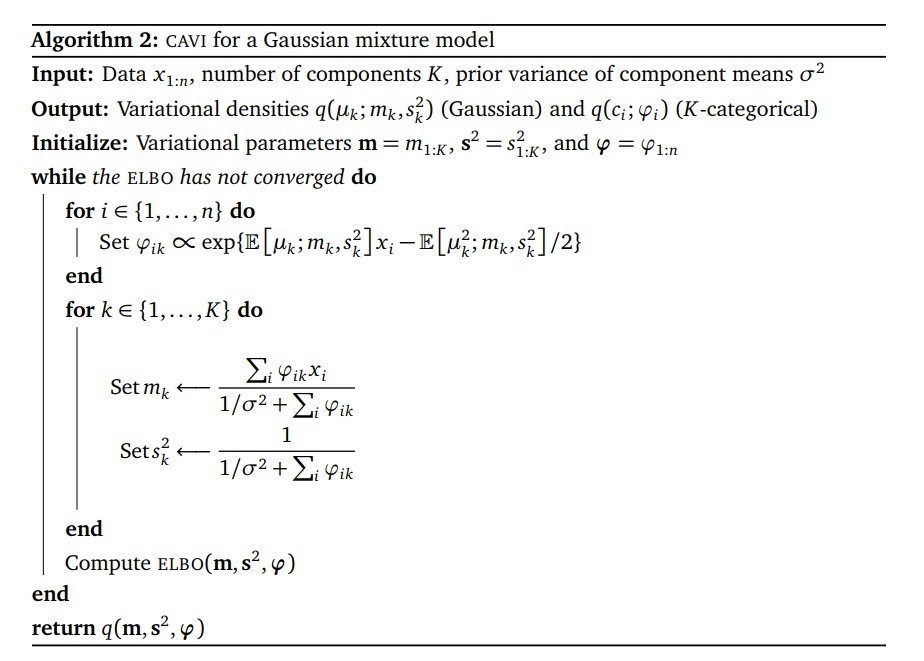# Variational Inference

Posted by Gwan Siu on March 29, 2018

## 1. What’s variational inference?

Representation, learning, and inference are 3 cores problem of machine learning. For statistic inference, it involves finding the approxiate model and parameters to represent the distribution given observed variables. In other work, given complete data $x$ and $y$ and unknown parameter $\theta$, this is classical parameter estimation problem in ML area. Usually, we adopt maximum likelihood estimation(MLE):

$\begin{equation} \theta^{\ast}=\arg\max_{\theta}p(x\vert y;\theta) \end{equation}$

in many real situations, we are given imcomplete data, i.e., only data $x$, in this case, latent variables $z$ are introduced. For example, in gassian mixture model, we introduce $z_{i}$ to indicate the underlying gaussian distribution. Thus, the overall formulation is changed

$\begin{equation} \displaystyle{p(x;\theta)=\int_{z}p(x,z;\theta)\mathrm{d}z} \end{equation}$

in fact, this integration usually is high-dimensional integration, which is intractable. It means that extract inference is impossible in this case. Therefore, we need to introduce approximate inference techniques in this case. Samling-based algorithms and variation-based algorithms are two kinds of approximate inference algorithms in modern bayesian statistics.

In this article, we mainly focus on variational inference(VI). The core idea of VI is to posit a family of distribution and then to find the member of that family which is close to the target, where closeness is measured using the Kullback-Leibler divergence.

In my previous article-EM, we can see that data likelihood can be decomposed into evidence lower bound and KL divergence:

$\begin{equation} \mathcal{L}(\theta)=\mathcal{F}(q,\theta)+\text{KL}(q(z),p(z\vert x;\theta)) \end{equation}$

where $\mathcal{F}(q,\theta)$ is evidence lower bound for marginal likelihood due to $\text{KL}(q(z),p(z\vert x;\theta))$ is non-negative.

$\begin{equation} \mathcal{L}(\theta)\geq \mathcal{F}(q,\theta) \end{equation}$

Instead of maximize marginal likelihood directly, EM algorithm and variational inference maximize the lower bound.

\begin{align} \mathcal{F}(q,\theta) &= \displaystyle{\int q(z)\ln\frac{p(x,z;\theta)}{q(z)}\mathrm{d}z} \\ &=\mathbb{E}_{q(z)}\big[\ln\frac{p(x,z;\theta)}{q(z)}\big] \\ &=\mathbb{E}_{q(z)}\big[\ln\frac{p(x\vert z;\theta)p(z;\theta)}{q(z)}\big] \\ &=\mathbb{E}_{q(z)}\big[\ln p(x\vert z)\big] - \text{KL}\big(q(z),p(z;\theta)\big) \end{align}
1. The first term is the expectation of the data likelihood and thus $\mathcal{F}(q,\theta)$ encourage distributions put their mass on configurations of latent variables that explain observed data.
2. The second term is the negative KL divergence between the variational distribution and the prior, so the $\mathcal{F}(q,\theta)$ force $q(z)$ to close to the prior $p(z)$.

Hence, maximize $\mathcal{F}(q,\theta)$ ** means to balance the likelihood and prior.**

## 2. Expectation-Maximization

In EM framework, we assume $q(z)=p(z\vert x;\theta^{old})$. The ELBO becomes:

\begin{align} \mathcal{F}(q,\theta) &= \displaystyle{\int q(z)\ln\frac{p(x,z;\theta)}{q(z)}\mathrm{d}z} \\ &= \displaystyle{\int q(z)\ln p(x,z;\theta)\mathrm{d}z - \int q(z)\ln q(z)} \\ &= \displaystyle{\int p(z\vert x;\theta^{old})\ln p(x,z;\theta)\mathrm{d}z - \int p(z\vert x;\theta^{old})\ln p(z\vert x;\theta^{old})} \\ &= Q(\theta, \theta^{old})-H(q) \end{align}

where $H(q)$ is the entropy of $z$ given $x$. It is constant w.r.t $\theta$ and thus we will not take it into account when we maximize ELBO. The EM algorithm is sufficient to maximize $Q(\theta, \theta^{old})$

E-step: maximize $\mathcal{F}(q,\theta)$ w.r.t distribution over hidden variables given the parameters:

\begin{align} &q^{(t+1)} =\arg\max_{q(z)} \mathcal{F}(q(z), \theta^{(t)}) \\ &\rightarrow p(z\vert x;\theta^{old}) \end{align}

M-step: maximize $\mathcal{F}(q,\theta)$ w.r.t the parameters given the hidden distribution

$\begin{equation} \int p(z\vert x;\theta^{old})\ln p(x,z;\theta)\mathrm{d}z \end{equation}$

## 3. Mean Field Theory

In EM framework, $q(z)=p(z\vert x;\theta^{old})$ is computed by iterative method. It means that we can find a analytical solution of $p(x\vert x;\theta^{old})$, this is possible for simple modles but can not be generalized to complex models. Instead, we approximate the posterior distribuiton by a family of simple dsitributions.

$\begin{equation} q(z) = \prod_{j=1}^{m}q(z_{j}) \end{equation}$

we assume the latent variables are mutually independent and each governed by a distinct factor in the variational distribution， i.e. $z_{i}\perp z_{j}$, for $i\neq j$. This is called mean-field theory.

## 4. Coodinate Ascent Variational Inference(CAVI)

In this part, I will compbined with mean-field theory and talk about how ELBO is maximize. One latent variabe posterior $q(z_{i})$ is updated by the rest latent variables $i\neq j$. Here, I will talk about CAVI algorithm. Let $q(z)=\prod_{i}q(z_{i})$. Then, the EBLO becomes:

\begin{align} \mathcal{F}(q,\theta) &= \displaystyle{\int q(z)\ln\frac{p(x,z;\theta)}{q(z)}\mathrm{d}z} \\ &= \displaystyle{\int \prod_{i} q(z_{i}) \ln p(x,z;\theta) \mathrm{d}z-\int \prod_{i} q(z_{i}) \ln q(z)\mathrm{d}z} \\ &= \displaystyle{\int q(z_{j}) \int \prod_{i\neq j}q(z_{i})\ln p(x,z;\theta) \prod_{i\neq j}\mathrm{d}z_{i}\mathrm{d}z_{j}- \sum_{i\neq j}\int q(z_{i})\ln q(z_{i})\mathrm{d}z_{i} - \int q(z_{j})\ln q(z_{j})\mathrm{d}z_{j}} \\ &=\int q_{j}\ln (\frac{\text{exp}(\mathbb{E}[\ln p(x,z;\theta)])}{q(z_{j})})\mathrm{d}z_{j}-\sum_{i\neq j}\int q(z_{i})\ln q(z_{i})\mathrm{d}z_{i} - \int q(z_{j})\ln q(z_{j})\mathrm{d}z_{j} \\ &=\dislaystyle{\int q(z_{j}\ln \frac{\hat{p}(z_{i\neq j})}{q(z_{j})})\mathrm{d}z_{j}+H(z_{i\neq j})} +c \\ &=-\text{KL}(q(z_{j}),\hat{p}(z_{i\neq j}))+H(z_{i\neq j}) + c \end{align}

Since KL divergence is non-negative, thus, ELBO is maximized when $\text{KL}(q(z_{j}),\hat{p}(z_{i\neq j}))=0$, i.e.

$\begin{equation} q(z_{j}) = \hat{p}(z_{i\neq j})= \frac{1}{Z}\text{exp}(\mathbb{E}[\ln p(x,z;\theta)]_{i\neq j}) \end{equation}$

Similarly, in variational EM:

E-step: $q^{\ast}=\frac{1}{Z}\text{exp}(\mathbb{E}[\ln p(x,z;\theta)]_{i\neq j})$

$\begin{equation} q(z) = \prod_{i}q(z_{i}) \end{equation}$

M-step: maximize the $\mathcal{F}(q,\theta)$.

The figure below is the process of CAVI algorithm:## 4. Variational inference and GMM

In this section, CAVI algorithm is used for Mixture of Gaussians model(GMM). It will be helpful to understand how CAVI works.

### 4.1 Joint distribution computation

Given observed data $X=(x_{1},…,x_{n})$ from $K$ independent gaussian distribution with mean $\mu_{k}$. One-hot vector $c_{i}\in \mathbb{R}^{k}$ indicate the distribution to which each data belong. The hyperparameter $\sigma^{2}$ is fixed. latent variables are $\mu, c$. The prior is:

\begin{align} \mu_{k} \sim \mathcal{N}(0, \sigma^{2}) \\ c_{i} \sim Categorical(\frac{1}{K},...,\frac{1}{K}) \\ x_{i} \sim \mathcal{N}(c_{i}^{T}, \mu, 1) \end{align}

According to bay theroem, we can compute the joint distribution:

\begin{align} p(\mu, c,x) &= p(\mu)p(c,x\vert \mu) \\ &= p(\mu)p(c)p(x\vert c, \mu) \\ &=p(\mu)\prod_{i=1}^{n}p(c_{i})p(x_{i}\vert c_{i}, \mu) \end{align}

once we have joint distribution, we can compute marginal distribution. However, the formulation has no analytical solution, and the computational complexity is $\mathcal{O}(K^{n})$.

\begin{align} p(x) &= \int \sum_{c}p(\mu, c, x)\mathrm{d}\mu \\ &= \int p(\mu)\prod_{i=1}^{n}\sum_{c_{i}}p(c_{i})p(x_{i}\vert c_{i}, \mu)\mathrm{d}\mu \end{align}

### 4.2 GMM and CAVI

Now, we should compute variational ditribution $q(z)$, where $m=(m_{1},…,m_{k}), s^{2}=(s_{1}^{2},…,s_{K}^{2}), \phi=(\phi_{1},…,\phi_{n})$ are variational parameters, hence the formulation of variational distribution is:

\begin{align} q(z) &= q(\mu,c) \\ &= \prod_{k=1}^{K}q(\mu_{k};m_{k},s_{k}^{2})\cdot \prod_{i=1}^{n}q(c_{i};\phi_{i}) \end{align}
1. we can obtain the formulation $\mathrm{ELBO}$, which is a function of $m,s^{2},\phi$.
\begin{align} \mathrm{ELBO}(q) &= \mathrm{ELBO}(m, s^{2}, \phi) \\ &= \mathbb{E}_{q(z)}[\log p(x\vert z)] + \mathbb{E}_{q(z)}[\log p(z)]-\mathbb{E}_{q(z)}[\log q(z)] \\ &= \sum_{i=1}^{n} \mathbb{E}[\log p(x_{i}\vert c_{i}, \mu;\phi_{i}, m, s^{2})] +(\sum_{k=1}^{K}\mathbb{E}[\log p(\mu_{k};m_{k},s^{2}_{k})]+\sum_{i=1}^{n}\mathbb{E}[\log p(c_{i};\phi_{i})]) \\ &- (\sum_{k=1}^{K}\mathbb{E}[\log q(\mu_{k};m_{k}, s_{k}^{2})]+\sum_{i=1}^{n}\mathbb{E}[\log q(c_{i};\phi_{i}]) \end{align}
1. from section 3, we obtain how CAVI algorithm update latent variables. Now, we applyied it into GMM to compute cluster indicator $c$ and update $c$, noted $\mu$ is fixed:
\begin{align} q^{\ast}_{j} &\propto exp(\mathbb{E}_{\mu}[\log p(c_{i}, \mu, x_{i})]) \\ &\propto exp(\mathbb{E}[\log p(x_{i}\vert c_{i}, \mu)\cdot \log p(c_{i},\mu)]) \\ &\propto exp(\mathbb{E}_{\mu}[\log p(x_{i}\vert c_{i}, \mu)]+\mathbb{E}_{\mu}[\log p(c_{i}, \mu)]) \\ &\propto exp(\mathbb{E}_{\mu}[\log p(c_{i}\vert c_{i},\mu)]+\log p(c_{i})) \end{align}

the second term $\log p(c_{i})$ is log prior and it is a constant. Hence, we pay our attention to the first term: the distribution of $c_{i}$ gaussian distribution. In detail, we simplify it due to $c_{i}=(c_{i1},…,c_{ik})$ is one-hot vector, and we have:

\begin{align} \mathbb{E}[\log p(x_{i}\vert c_{i},\mu)] &= \sum_{k}c_{ik}\mathbb{E}_{\mu_{k}}[\log p(x_{i}\vert \mu_{k})] \\ &= \sum_{k}c_{ik}\mathbb{E}_{\mu_{k}}[-\frac{(x-\mu_{k})^{2}}{2}]+const \\ &= \sum_{k}c_{ik}(\mathbb{E}_{\mu_{k}}[\mu_{k}]x_{i}+\mathbb{E}_{\mu_{k}}[\mu_{k}^{2}]/2) + const \end{align}

from the formulation above, $\mathbb{E}[\mu_{k}]$ and $\mathbb{E}[\mu_{k}^{2}]$ can be computed. For each data point $i$, parameter $\phi_{ik}$ in the $k$th component of the latent variable $c$. The updated formulation is:

$\begin{equation} \phi_{ik}\propto exp(\mathbb{E}[\mu_{k}]x_{i}+\mathbb{E}[\mu_{k}^{2}]/2) \end{equation}$

smiliarly, we can compute latent variable $\mu$ of GMM. Firstly, we should calculate the optimal variational distribution $q(\mu_{k})$, and the update the parameter $m_{k}, s_{k}^{2}$ of $\mu_{k}$:

\begin{align} m_{k} &= \frac{\sum_{i}\phi_{ik}\cdot x_{i}}{1/\sigma^{2}+\sum_{i}\phi_{ik}} \\ s_{k}^{2} &= \frac{1}{1/\sigma^{2}+\sum_{i}\phi_{ik}} \end{align}

The algorithm of GMM and CAVI is below:## 5. Comparision of MCMC and VI

MCMC VI
More computationally intensive Less intensive
Gaurantess producing asymptotically exact samples from the target distribution No such gaurantees
Slower Faster, expecially for large data sets and complex distributions
Best for precise inference Useful to explore many scenarios quickly or large data sets

Reference